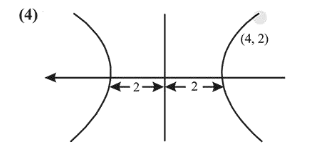# A hyperbola has its centre at the origin,Question:

A hyperbola has its centre at the origin, passes through the point $(4,2)$ and has transverse axis of length 4 along the $x$-axis. Then the eccentricity of the hyperbola is :

1. (1) $\frac{3}{2}$

2. (2) $\sqrt{3}$

3. (3) 2

4. (4) $\frac{2}{\sqrt{3}}$

Correct Option: , 4

Solution:Consider equation of hyperbola

$\frac{x^{2}}{2^{2}}-\frac{y^{2}}{b^{2}}=1$

$\because(4,2)$ lies on hyperbola

$\therefore \frac{16}{4}-\frac{4}{b^{2}}=1$

$\therefore b^{2}=\frac{4}{3}$Since, eccentricity $=\sqrt{1+\frac{b^{2}}{a^{2}}}$

Hence, eccentricity $=\sqrt{1+\frac{\frac{4}{3}}{4}}=\sqrt{1+\frac{1}{3}}=\frac{2}{\sqrt{3}}$